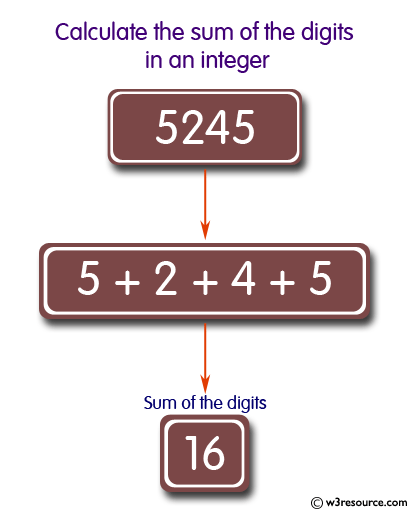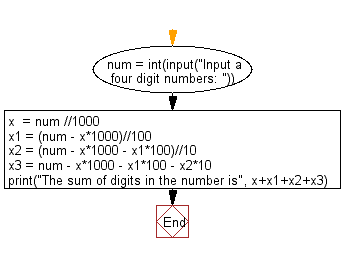﻿ Python: Calculate sum of digits of a number - w3resource# Python: Calculate sum of digits of a number

## Python Basic: Exercise-68 with Solution

Write a Python program to calculate sum of digits of a number.

Pictorial Presentation:Sample Solution:-

Python Code:

``````num = int(input("Input a four digit numbers: "))
x  = num //1000
x1 = (num - x*1000)//100
x2 = (num - x*1000 - x1*100)//10
x3 = num - x*1000 - x1*100 - x2*10
print("The sum of digits in the number is", x+x1+x2+x3)
```
```

Sample Output:

```Input a four digit numbers: 5245
The sum of digits in the number is 16
```

Flowchart:## Visualize Python code execution:

The following tool visualize what the computer is doing step-by-step as it executes the said program:

Python Code Editor:

Have another way to solve this solution? Contribute your code (and comments) through Disqus.

What is the difficulty level of this exercise?

Test your Programming skills with w3resource's quiz.

﻿

## Python: Tips of the Day

Join Strings in an Iterable:

```>>> words = ('Hello', 'Python', 'Programmers')
>>> '!'.join(words)
'Hello!Python!Programmers'
>>> words_dict = {0: 'zero', 1: 'one', 2: 'two', 3: 'three'}
>>> '&'.join(words_dict.values())
'zero&one&two&three'
```